## IDAPython 让你的生活更滋润 part1 and part2

2016-01-06 09:11:00

# 0x01 背景

• Chris Eagle的The IDA Pro Book
• Alex Hanel的The Beginner’s Guide to IDAPython
• Magic Lantern 的IDAPython Wiki

# 0x02 利用IDAPython解决字符串加密问题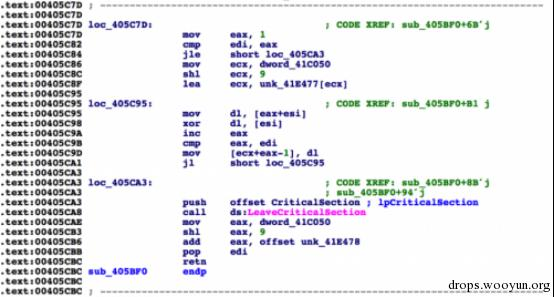图片1 字符串解密函数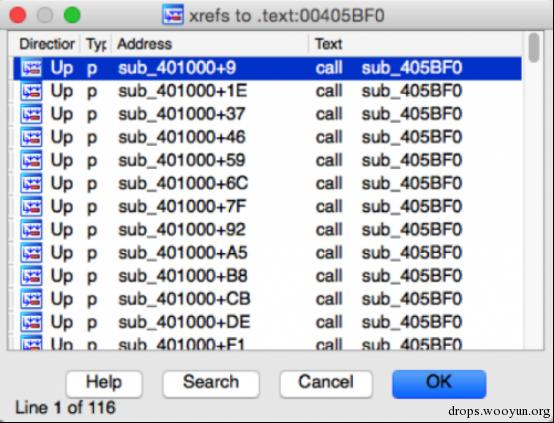图2 大量的对可疑函数的引用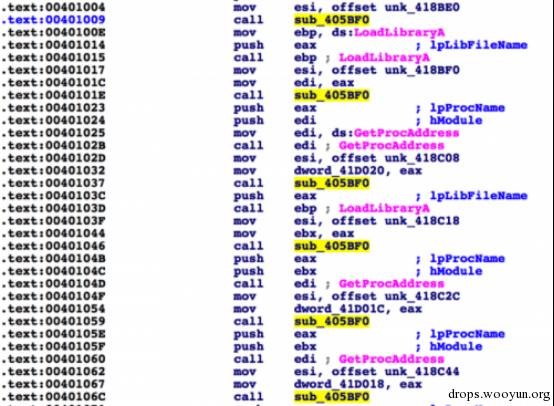被调用的实例

1. 我可以手动解密然后重命名这些字符串。
2. 我可以动态调试这个样本然后重命名遇到的字符串
3. 我可以写一个脚本用来解密并且重命名这些字符串

E4 91 96 88 89 8B 8A CA 80 88 88

``````def decrypt(data):
length = len(data)
c = 1
o = ""
while c < length:
o += chr(ord(data) ^ ord(data[c][/c]))
c += 1
return o
``````

``````>>> from binascii import *
>>> d = unhexlify("E4 91 96 88 89 8B 8A CA 80 88 88".replace(" ",''))
>>> decrypt(d)
'urlmon.dll'
``````

``````for addr in XrefsTo(0x00405BF0, flags=0):

Result:
0x401009L
0x40101eL
0x401037L
0x401046L
0x401059L
0x40106cL
0x40107fL
<truncated>
``````

``````def find_function_arg(addr):
while True:
if GetMnem(addr) == "mov" and "esi" in GetOpnd(addr, 0):
print “We found it at 0x%x” % GetOperandValue(addr, 1)
break

Example Results:
Python>find_function_arg(0x00401009)
We found it at 0x418be0
``````

``````def get_string(addr):
out = ""
while True:
if Byte(addr) != 0:
else:
break
return out
``````

``````def find_function_arg(addr):
while True:
if GetMnem(addr) == "mov" and "esi" in GetOpnd(addr, 0):
return ""

out = ""
while True:
if Byte(addr) != 0:
else:
break
return out

def decrypt(data):
length = len(data)
c = 1
o = ""
while c < length:
o += chr(ord(data) ^ ord(data[c][/c]))
c += 1
return o

print "[*] Attempting to decrypt strings in malware"
for x in XrefsTo(0x00405BF0, flags=0):
ref = find_function_arg(x.frm)
string = get_string(ref)
dec = decrypt(string)
print "Ref Addr: 0x%x | Decrypted: %s" % (x.frm, dec)

Results:
[*] Attempting to decrypt strings in malware
Ref Addr: 0x401009 | Decrypted: urlmon.dll
Ref Addr: 0x401037 | Decrypted: wininet.dll
Ref Addr: 0x401046 | Decrypted: InternetOpenA
Ref Addr: 0x401059 | Decrypted: InternetOpenUrlA
<truncated>
``````

``````MakeComm(x.frm, dec)
MakeComm(ref, dec)
``````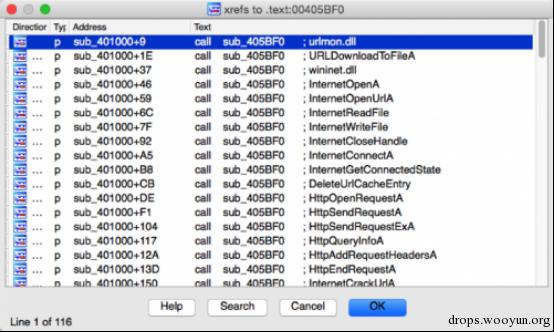图4 运行完脚本后的字符串交叉引用界面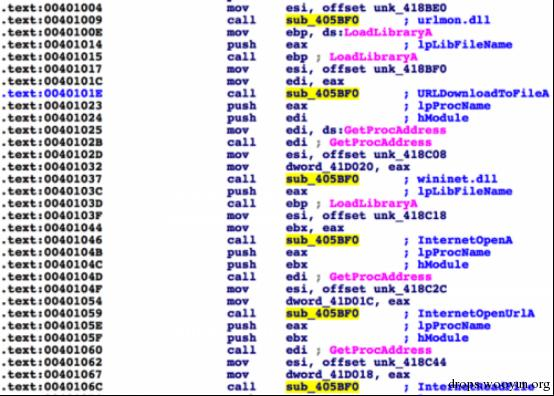图5 运行完脚本后的反汇编代码

# 0x03 利用IDAPython解决函数/库调用的哈希混淆问题图片6 malware使用CRC32哈希算法来动态的加载函数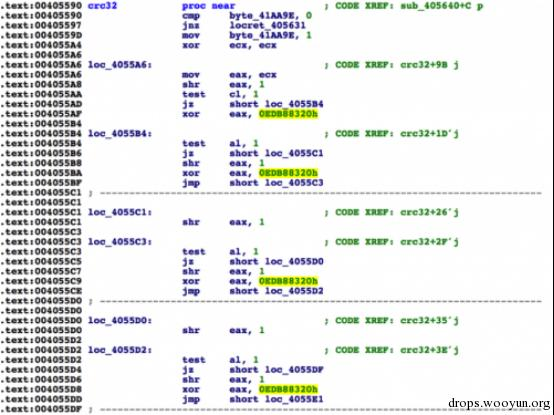图片7 确认CRC32算法

``````def get_functions(dll_path):
pe = pefile.PE(dll_path)
if ((not hasattr(pe, 'DIRECTORY_ENTRY_EXPORT')) or (pe.DIRECTORY_ENTRY_EXPORT is None)):
print "[*] No exports for %s" % dll_path
return []
else:
expname = []
for exp in pe.DIRECTORY_ENTRY_EXPORT.symbols:
if exp.name:
expname.append(exp.name)
return expname
``````

``````def calc_crc32(string):
return int(binascii.crc32(string) & 0xFFFFFFFF)
``````

``````HASH => NAME
``````

https://github.com/pan-unit42/public_tools/blob/master/ida_scripts/gen_function_json.py

``````for k,v in json_data.iteritems():
json_data[int(k)] = json_data.pop(k)
``````

http://www.cprogramming.com/tutorial/enum.html

``````enumeration = GetEnum("crc32_functions")
if enumeration == 0xFFFFFFFF:
enumeration = AddEnum(0, "crc32_functions", idaapi.hexflag())
``````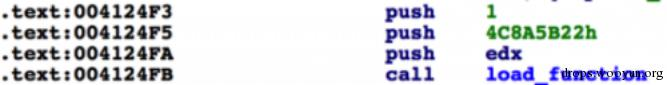的参数

``````for x in XrefsTo(load_function_address, flags=0):
push_count = 0
if GetMnem(current_address) == "push":
push_count += 1
data = GetOperandValue(current_address, 0)
if push_count == 2:
if data in json_data:
name = json_data[data]
``````

``````AddConstEx(enumeration, str(name), int(data), -1)
``````

``````def get_enum(constant):
all_enums = GetEnumQty()
for i in range(0, all_enums):
enum_id = GetnEnum(i)
enum_constant = GetFirstConst(enum_id, -1)
name = GetConstName(GetConstEx(enum_id, enum_constant, 0, -1))
if int(enum_constant) == constant: return [name, enum_id]
while True:
enum_constant = GetNextConst(enum_id, enum_constant, -1)
name = GetConstName(GetConstEx(enum_id, enum_constant, 0, -1))
if enum_constant == 0xFFFFFFFF:
break
if int(enum_constant) == constant: return [name, enum_id]
return None

constant = GetOperandValue(addr, 0)
enum_data = get_enum(constant)
if enum_data:
name, enum_id = enum_data
OpEnumEx(addr, 0, enum_id, 0)
return True
else:
return False
``````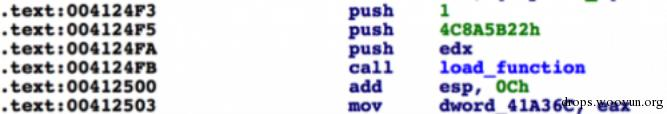图片9 当加载完函数后，程序将函数地址存储到了DWORD地址

``````address = current_address
if GetMnem(address) == "mov":
if 'dword' in GetOpnd(address, 0) and 'eax' in GetOpnd(address, 1):
operand_value = GetOperandValue(address, 0)
MakeName(operand_value, str("d_"+name))
``````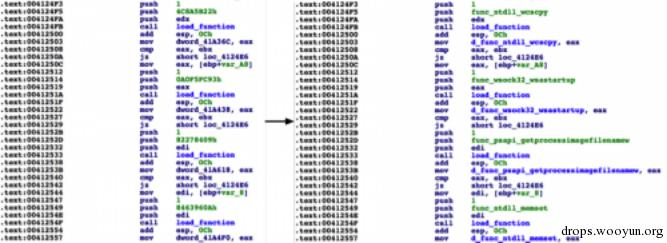图片10 运行完脚本后的变化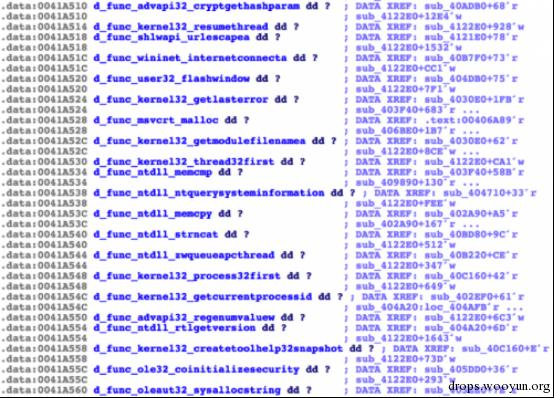``````import json

def get_enum(constant):
all_enums = GetEnumQty()
for i in range(0, all_enums):
enum_id = GetnEnum(i)
enum_constant = GetFirstConst(enum_id, -1)
name = GetConstName(GetConstEx(enum_id, enum_constant, 0, -1))
if int(enum_constant) == constant: return [name, enum_id]
while True:
enum_constant = GetNextConst(enum_id, enum_constant, -1)
name = GetConstName(GetConstEx(enum_id, enum_constant, 0, -1))
if enum_constant == 0xFFFFFFFF:
break
if int(enum_constant) == constant: return [name, enum_id]
return None

constant = GetOperandValue(addr, 0)
enum_data = get_enum(constant)
if enum_data:
name, enum_id = enum_data
OpEnumEx(addr, 0, enum_id, 0)
return True
else:
return False

push_count = 0
if GetMnem(current_address) == "push":
push_count += 1
data = GetOperandValue(current_address, 0)
if push_count == 2:
if data in json_data:
name = json_data[data]
AddConstEx(enumeration, str(name), int(data), -1)
print "[+] Converted 0x%x to %s enumeration" % (current_address, name)
if GetMnem(address) == "mov":
if 'dword' in GetOpnd(address, 0) and 'eax' in GetOpnd(address, 1):
operand_value = GetOperandValue(address, 0)
MakeName(operand_value, str("d_"+name))

fh = open("output.json", 'rb')
fh.close()

# JSON objects don't allow using integers as dict keys. Little workaround for
# this issue.
for k,v in json_data.iteritems():
json_data[int(k)] = json_data.pop(k)

conversion_function = 0x00405680
enumeration = GetEnum("crc32_functions")
if enumeration == 0xFFFFFFFF:
enumeration = AddEnum(0, "crc32_functions", idaapi.hexflag())
enum_for_xrefs(conversion_function, json_data, enumeration)
``````

# 0x04 总结

### 路

2016-01-06 13:43:54

### 路

2016-01-06 14:33:56

### 路

2016-01-06 15:18:58

### F

2016-01-07 16:10:59

### Z

2016-01-09 13:41:43

### 路

2016-01-10 14:23:49

### 寂

2016-01-26 09:01:28#### 蒸米PHP安全 文章：45 篇

iOS安全 文章：36 篇

### 扫码关注公众号### D

#### Da22le0rz

### U

#### Uesaka